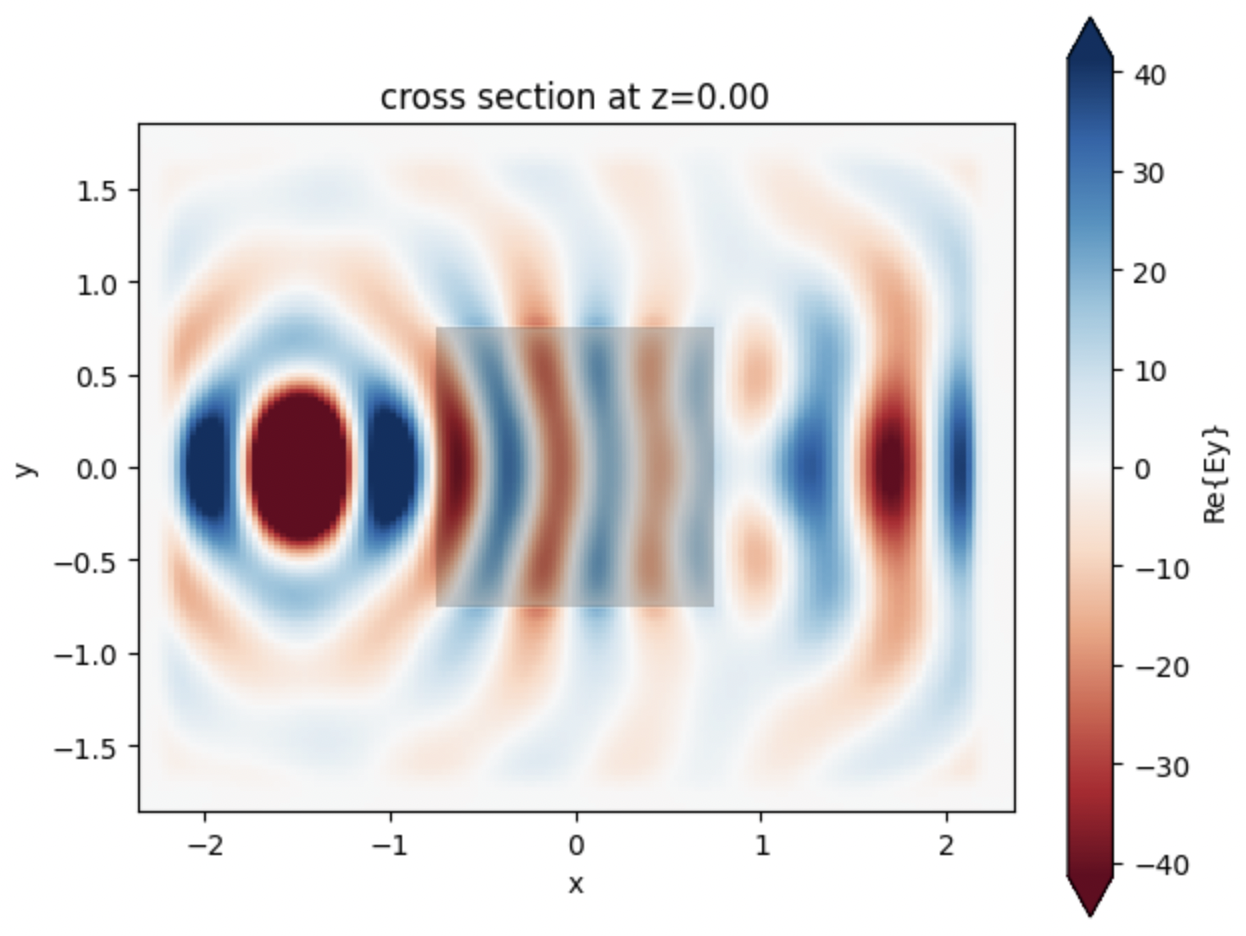# Tidy3D Documentation#

Tidy3D is a software package for solving extremely large electrodynamics problems using the finite-difference time-domain (FDTD) method. It can be controlled through either an open source python package or a web-based graphical user interface.

If you do not wish to install, please click this button to get started quickly.

## 1. Set up Tidy3D#

Install the python library tidy3d for creating, managing, and postprocessing simulations with

```pip install tidy3d
```

Next, configure your tidy3d package with the API key from your account.

```tidy3d configure
```

And enter your API key when prompted.

## 2. Run a Simulation#

Start running simulations with just a few lines of code. Run this sample code to simulate a 3D dielectric box in Tidy3D and plot the corresponding field pattern.

```# !pip install tidy3d # if needed, install tidy3d in a notebook by uncommenting this line

# import the tidy3d package and configure it with your API key
import numpy as np
import tidy3d as td
import tidy3d.web as web
# web.configure("YOUR API KEY") # if authentication needed, uncomment this line and paste your API key here

# set up global parameters of simulation ( speed of light / wavelength in micron )
freq0 = td.C_0 / 0.75

# create structure - a box centered at 0, 0, 0 with a size of 1.5 micron and permittivity of 2
square = td.Structure(
geometry=td.Box(center=(0, 0, 0), size=(1.5, 1.5, 1.5)),
medium=td.Medium(permittivity=2.0)
)

# create source - A point dipole source with frequency freq0 on the left side of the domain
source = td.PointDipole(
center=(-1.5, 0, 0),
source_time=td.GaussianPulse(freq0=freq0, fwidth=freq0 / 10.0),
polarization="Ey",
)

# create monitor - Measures electromagnetic fields within the entire domain at z=0
monitor = td.FieldMonitor(
size=(td.inf, td.inf, 0),
freqs=[freq0],
name="fields",
colocate=True,
)

# Initialize simulation - Combine all objects together into a single specification to run
sim = td.Simulation(
size=(4, 3, 3),
grid_spec=td.GridSpec.auto(min_steps_per_wvl=25),
structures=[square],
sources=[source],
monitors=[monitor],
run_time=120/freq0,
)

print(f"simulation grid is shaped {sim.grid.num_cells} for {int(np.prod(sim.grid.num_cells)/1e6)} million cells.")

# run simulation through the cloud and plot the field data computed by the solver and stored in the monitor
data = td.web.run(sim, task_name="quickstart", path="data/data.hdf5", verbose=True)
ax = data.plot_field("fields", "Ey", z=0)
```

This will produce the following plot, which visualizes the electromagnetic fields on the central plane.## 3. Analyze Results#

1. Postprocess simulation data using the same python session, or

2. View the results of this simulation on our web-based graphical user interface.